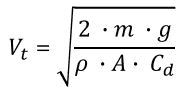# Terminal Velocity Calculator

Easily calculate the terminal velocity of a human or object falling through air with this calculator. Required inputs are: the mass of the falling object/body, the drag coefficient and the projected area, as well as the air density and gravitational acceleration. Works well for skydiving jumps.

Share calculator:

Embed this tool:
get code

## What is Terminal Velocity?

Terminal velocity is defined as the maximum velocity an object can achieve when falling through a fluid, such as air or water. That happens when the gravitational force working on the object in downward direction equals the sum of upward forces (drag and buoyancy) impeding it's fall. An object moving at terminal velocity has zero acceleration and constant speed as the net force on it is zero by definition.

### Terminal Velocity of a Human

The terminal velocity of an average 80 kg human body is about 66 meters per second (= 240 km/h = 216 ft/s = 148 mph). Terminal velocity can be achieved by an object provided it has enough distance to fall through so if you want to experience it, you need to jump from a high enough place (do not forget your parachute!). For example, a human body generally needs to fall about 450 meters (1,500 feet) of height before it reaches terminal velocity. Such a fall takes roughly 12 seconds.

## Terminal Velocity Formula

The formula for the terminal velocity of a falling object (Vt) can be calculated from the body's mass m, the density of the fluid in question (p, in kg/m3, e.g. 1.225 for air), the cross-sectional area projected by the object (A), and the gravitational (or equivalent) force g in m/s2 according to the following equation:This equation applies only for objects falling through air or in other cases where the buoyancy force is negligible due to the large difference between the density of the fluid and the falling object (e.g. ~1.2 kg/m3 for air versus 985 kg/m3 for the human body). In our calculator you can enter gravity both in m/s2 and as g-units where 1g = 9.80665 m/s2 is the standard acceleration due to Earth's gravity at sea-level.

The drag coefficient is undoubtedly the hardest thing to estimate in the terminal velocity calculator input. Its value is determined empirically, usually with the use of a wind tunnel. Some example drag coefficients are 1.0 for a cube or a skydiver falling flat on his belly, 0.5 for a sphere and 0.04 for an aerodynamic wing. A coefficient of drag of 0.294 should work relatively well for a human body falling head first whereas feet first it should be around 0.70 . The formula only works well if the drag coefficient was determined for speeds of similar magnitude and if it doesn't change a lot during the fall. One has to be careful when applying drag coefficients calculated at, say, winds under 30 m/s winds for airflow near and faster than the speed of sound. At such speeds there is a large increase in the drag coefficient because of the formation of shock waves on the object so either a different coefficient should be used, or a coefficient which compensates for compressibility effects.

The terminal velocity equation tells us that an object with a large cross-sectional area or high drag coefficient would fall more slowly than an equivalent object with a smaller area or lower drag coefficient. If a skydiver spreads their hands in the area they would fall slower than if they curl into a ball or drop head-first or feet-first. It also says that all else being equal, a lighter object has a lower terminal velocity since it takes less time for the force of gravity to be balanced by the air resistance / drag force. You can check this by using different drag coefficiencts and body mass values in the terminal velocity calculator above to explore these relationships. They are the reason parachutes work: they vastly increase the cross-sectional area while their form is such that it significantly increases the coefficient of drag.

## Calculation examples

Example 1: A cannon ball with a radius of 30 cm and 20 kg weight is dropped from a plane cruising at high altitude. What is the terminal velocity such a cannon ball can achieve?

Let us assume the cannon ball is nearly a perfect sphere. We know that the coefficient of drag for a sphere is about 0.5. Taking the air density to be 1.225 kg/m3 and the gravity to be 1 g = 9.80665 m/s2 we only need to calculate the ball's projected area A before substituting in the terminal velocity equation above. By using our area of a circle calculator we can easily compute the area to be 1,256 cm2 or 0.1256 m2. Substituting in the terminal velocity formula we find Vt = √(2·20·9.80665 / (1.225·0.1256·0.5)) = √(392.266/0.07693) = √5099 = 71 m/s (233 ft/s). If we compare this result with the terminal velocity of a 80kg human we can see that the ball is falling somewhat faster even though it has 4x less mass and a higher coefficient of drag, which is due to it's ~8 times smaller projected area.

Example 2: An 80 kg skydiver is plunging towards the Earth face down and has already achieved terminal velocity of 66 m/s with his current cross-sectional area of 1m2 and a drag coefficient of about 1.00. If he changes his position to head first, thus reducing his cross-sectional area to only 0.5 m2 and his coefficient of drag becomes 0.25, what is the new terminal velocity he can achieve?

Plugging in the numbers we get the new terminal velocity to be around 101 m/s or 364 km/h. This is still way short of the world record for the fastest skydive: ~373 m/s.The above examples are just educational ones, obviously. Pleas, note that our terminal velocity calculator will automatically convert for you any units to the base SI units.